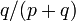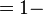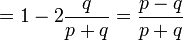## Wednesday, 24 December 2014

### A Pretty Little Voting Result in Combinatorics

I was updating the Bio for  Joseph Louis François Bertrand for my On This Day in Math pages, (see Births, 1822) and was reminded of the beautiful simplicity (and symmetry) hiding in this old problem.  Bertrand gave the problem, and a proof, in 1887 in J. Bertrand, Solution d'un problème, Comptes Rendus de l'Académie des Sciences.

The problem can be simply stated in a couple of ways, but Betrand's question was along the order of, " If at the end of an election, A had m votes and B had n votes, with m greater than n, what is the probability that at any point in the counting A had more votes than B.
In a second variation one might ask for the probability that A was never behind, thus including sequences that include one or more possible ties in the vote counts.

For those who want to sit and work on the problem for awhile, I will talk a little about the subsequent history of the problem, and give a solution to two variations much farther down.

In Bertrand's original paper, he sketches a proof based on a general formula for the number of favorable sequences using a recursion relation. He remarks that it seems probable that such a simple result could be proved by a more direct method. Such a proof was given by Désiré André based on the observation that the unfavorable sequences can be divided into two equally probable cases, one of which (the case where B receives the first vote) is easily computed; he proves the equality by an explicit bijection. A variation of his method is popularly known as André's reflection method, although André did not use any reflections. The first known use of reflections in the solution was by Percy MacMahon who applied theory of partitions to the problem. The most famous and elegant of these variations is the “reflection method” in which ballot permutations are represented as lattice paths and portions of the bad paths are reflected across a line.

Lost(and Found) in Translation: André’s ActualMethod and its Application to the Generalized Ballot Problem.  This article, by Marc Renault, appeared in the American Mathematical Monthly, April 2008.

The same author has also written the following which gives four different proofs of the general theorem, including some nice graphics.

Some steps and the Solution:

Renault gives a proof following these steps

How many ways can the  a + b  votes be arranged so that  A  maintains a lead throughout the counting of the ballots?
Here is the proof by reflection as given by the Wikipedia article on the problem, which also has several other proofs.

For A to be strictly ahead of B throughout the counting of the votes, there can be no ties. Separate the counting sequences according to the first vote. Any sequence that begins with a vote for B must reach a tie at some point, because A eventually wins. For any sequence that begins with A and reaches a tie, reflect the votes up to the point of the first tie (so any A becomes a B, and vice-versa) to obtain a sequence that begins with B. Hence every sequence that begins with A and reaches a tie is in one to one correspondence with a sequence that begins with B, and the probability that a sequence begins with B is$q/(p+q)$, so the probability that A always leads the vote is$= 1-$ the probability of sequences that tie at some point$= 1-$ the probability of sequences that tie at some point and begin with A or B$= 1-2\frac{q}{p+q}=\frac{p-q}{p+q}$